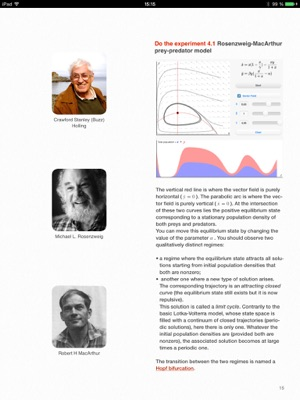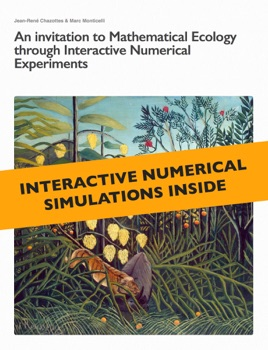## Description de l’éditeur

*** Interactive Numerical Experiments Inside ***

Summary :

The purpose of this booklet is to introduce some basic models in ecology based on differential equations. We assume little mathematical background. Readers need to know what the derivative of a function is.

This augmented booklet is the second one within a collection titled Experimentarium Digitale and dedicated to mathematical modeling (in physics, biology, etc).

A unique feature of these booklets is the interactive numerical experiments they contain and which allow the reader to play with the presented models.

Interactive Numerical Experiments of this booklet :

• Lotka-Volterra prey-predator model.

• Lotka-Volterra prey-predator model with competition among preys.

• Vector field and associated solutions.

• Rosenzweig-MacArthur model.

• Competion model.

• Logistic growth equation.

•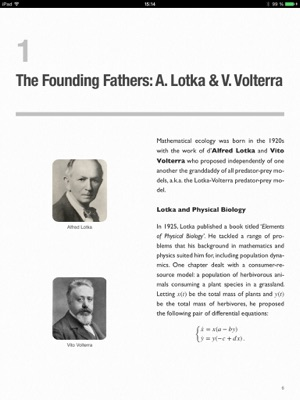•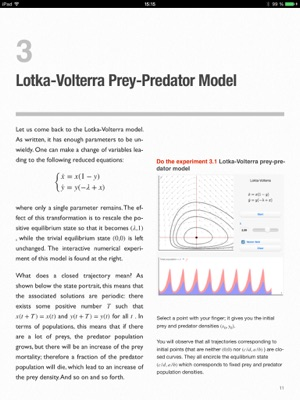•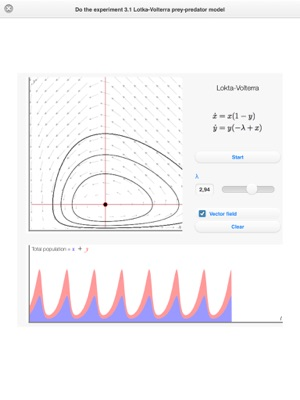•